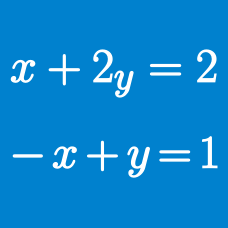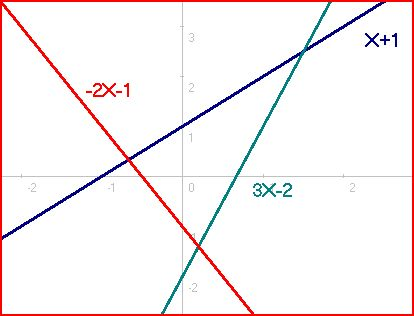Algebra

# System of Equations: Level 5 Challenges

$\begin{cases} 4\dfrac{\sqrt{x^{2} + 1}}{x} = 5\dfrac{\sqrt{y^{2} + 1}}{y}=6\dfrac{\sqrt{z^{2} + 1}}{z} \\ xyz = x + y + z\end{cases}$

If $$x = \dfrac{\sqrt{a}}{b} , y = c\dfrac{\sqrt{d}}{e} , z = f\sqrt{g}$$ satisfy the above system of equations, then find $$(a + b + \ldots + g )$$.

• The expression is in simplest terms, meaning that all of the variables are positive integers, $$a, d, g$$ are square free and $$c, e$$ are coprime.

Let $$a$$ and $$b$$ be positive integers such that $$a\ge\ b$$ and$\frac{a+1}{b}+\frac{b+1}{a}$is also a positive integer. Find sum of all possible values of $$a$$ which are less than 1000.The system of equations $4a^4+36a^2b^2+9b^4=20a^2+30b^2-1$ $2a^3b+3ab^3=5ab$ has $$n$$ nonnegative (meaning both $$a$$ and $$b$$ are nonnegative) real solutions. Let these solutions be $$(a_1,b_1),(a_2,b_2),\cdots (a_n,b_n)$$. The value of the sum $\sum_{i=1}^n a_i+b_i$ can be expressed in the form $$\dfrac{a+b\sqrt{c}}{d}$$ where $$\gcd (a,b,d)=1$$ and $$c$$ is squarefree. Find $$a+b+c+d$$.

A positive real number is given. In each move, we can either add $$3$$ to it, subtract $$3$$ to it, multiply it by $$3$$ and divide it by $$3$$. The sum of all numbers such that after exactly $$3$$ moves, the original number comes back is $$\frac{a}{b}$$, where $$a$$ and $$b$$ are relatively prime positive integers. Find $$a + b$$.

Find the largest possible value of $$x^3+y^3+z^3$$ for real $$x,\ y,\ z,$$ such that $\begin{cases} xyz^2=-64y-128x\\ x^2yz=-32y-32z\\ 3xy^2z=128x-64z \end{cases}$

×